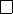4Matrices and linear equations

IA Vectors and Matrices4.4 Matrix rank
Consider a linear map
α
:
R
n
R
m
. Recall the rank
r
(
α
) is the dimension of
the image. Suppose that the matrix
A
is associated with the linear map. We
also call r(A) the rank of A.
Recall that if the standard basis is
e
1
, ···e
n
, then
Ae
1
, ··· , Ae
n
span the
image (but not necessarily linearly independent).
Further,
Ae
1
, ··· , Ae
n
are the columns of the matrix
A
. Hence
r
(
A
) is the
number of linearly independent columns.
Definition
(Column and row rank of linear map)
.
The column rank of a matrix
is the maximum number of linearly independent columns.
The row rank of a matrix is the maximum number of linearly independent
rows.
Theorem. The column rank and row rank are equal for any m × n matrix.
Proof.
Let
r
be the row rank of
A
. Write the biggest set of linearly independent
rows as
v
T
1
, v
T
2
, ···v
T
r
or in component form
v
T
k
= (
v
k1
, v
k2
, ··· , v
kn
) for
k
=
1, 2, ··· , r.
Now denote the ith row of A as r
T
i
= (A
i1
, A
i2
, ···A
in
).
Note that every row of
A
can be written as a linear combination of the
v
’s.
(If
r
i
cannot be written as a linear combination of the
v
’s, then it is independent
of the
v
’s and
v
is not the maximum collection of linearly independent rows)
Write
r
T
i
=
r
X
k=1
C
ik
v
T
k
.
For some coefficients C
ik
with 1 i m and 1 k r.
Now the elements of A are
A
ij
= (r
i
)
T
j
=
r
X
k=1
C
ik
(v
k
)
j
,
or
A
1j
A
2j
.
.
.
A
mj
=
r
X
k=1
v
k
j
C
1k
C
2k
.
.
.
C
mk
So every column of
A
can be written as a linear combination of the
r
column
vectors c
k
. Then the column rank of A r, the row rank of A.
Apply the same argument to
A
T
to see that the row rank is
the column
rank.